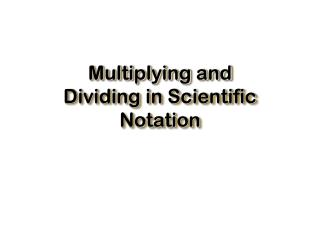DownloadDownload PresentationMultiplying and Dividing in Scientific Notation

# Multiplying and Dividing in Scientific Notation

Télécharger la présentation## Multiplying and Dividing in Scientific Notation

- - - - - - - - - - - - - - - - - - - - - - - - - - - E N D - - - - - - - - - - - - - - - - - - - - - - - - - - -
##### Presentation Transcript

1. Multiplying and Dividing in Scientific Notation

2. Multiplying Numbers in Scientific Notation • Multiply the base numbers together. • Then add the exponents to get the power of 10. • General formula: (N X 10x) (M X 10y) = (N) (M) X 10x+y

3. Example 1 • Given: (3.42 X 105)(4.67 X 109) • Multiply bases: 3.42 X 4.67 = 15.97 • Add Exponents: 105+109=105+9=1014 • Answer: 15.97 X 1014 • Shift Decimal left one place. • Final Answer: 1.597 X 1014

4. Example 2 • Given: (2.93 X 10-2)(8.3 X 10-5) • Multiply Bases: 2.93 X 8.3 = 24.319 • Add Exponents: 10-2+10-5=10-2+(-5)=10-7 • Answer: 24.319 X 10-7 • Shift decimal left one place. • Final Answer: 2.43 X 10-6

5. Practice Worksheet Practice Multiplying in Scientific Notation (2.4 X 10-3)(4.5 X 10-3) = (5.67 X 10^3)(3.9 X 10^-6) = (4.78 X 107)(9.3 X 10-2) = (8.9 X 10^-2)(6.5 X 10^4) = (3.4 X 108)(5 X 103) =

6. Practice Worksheet Practice Multiplying in Scientific Notation (2.4 X 10-3)(4.5 X 10-3) = 1.08 X 10-5 (5.67 X 10^3)(3.9 X 10^-6) = 2.21 X 10^-2 (4.78 X 107)(9.3 X 10-2) = 4.45 X 106 (8.9 X 10^-2)(6.5 X 10^4) = 5.785 X 10^3 (3.4 X 108)(5 X 103) = 1.7 X 1012

7. Practice Worksheet Practice Multiplying in Scientific Notation (1.6 X 10-6)(9.9 X 10-9) = (5.1 X 10^4)(9.1 X 10^2) = (2.0 X 10-3)(2.3 X 104) = (7.4 X 10^2)(6.5 X 10^7) = (9.4 X 10-5)(3.1 X 105) =

8. Practice Worksheet Practice Multiplying in Scientific Notation (1.6 X 10-6)(9.9 X 10-9) = 1.584 X 10-14 (5.1 X 10^4)(9.1 X 10^2) = 4.641 X 10^7 (2.0 X 10-3)(2.3 X 104) = 4.6 X 101 (7.4 X 10^2)(6.5 X 10^7) = 4.81 X 10^10 (9.4 X 10-5)(3.1 X 105) = 2.914 X 101

9. Dividing Numbers in Scientific Notation • Divide the base numbers. • Then subtract the exponents to get the power of 10. • General formula: N X 10x / M X 10y = N/M X 10x-y

10. Example 1 • Given:8 X 10-3 / 2 X 10-2 • Divide bases: 8/2=4 • Subtract exponents: (-3)-(-2)=-1 • Answer: 4 X 10-1

11. Example 2 • Given:3.45 X 102 / 1.23 X 10-5 • Divide bases: 3.45/1.23= 2.81 • Subtract exponents: (2)-(-5)=7 • Answer: 2.81 X 107

12. Practice Worksheet Practice Dividing in Scientific Notation 3.0 X 104 / 1.5 X 10-2 = 4.5 X 10^2 / 2.4 X 10^-5 = 4.9 X 105 / 1.45 X 108 = 6 X 10^5 / 3 X 10^-7 = 2.46 X 106 / 4.523 X 103 =

13. Practice Worksheet Practice Dividing in Scientific Notation 3.0 X 104 / 1.5 X 10-2 = 2.0 X 106 4.5 X 10^2 / 2.4 X 10^-5 = 1.875 X 10^7 4.9 X 105 / 1.45 X 108 = 3.37 X 10-3 6 X 10^5 / 3 X 10^-7 = 2 X 10^12 2.46 X 106 / 4.523 X 103 = 5.44 X 102

14. Practice Worksheet Practice Dividing in Scientific Notation 1.51 X 105 / 3.66 X 10-4 = 3.45 X 108 / 2.6 X 103 = 8.21 X 10^-5 / 4 X 10^3 = 4 X 10^2 / 2 X 10^-8 = 3.4 X 10-8 / 1.2 X 10-4 =

15. Practice Worksheet Practice Dividing in Scientific Notation 1.51 X 105 / 3.66 X 10-4 = 4.13 X 108 3.45 X 108 / 2.6 X 103 = 1.33 X 105 8.21 X 10^-5 / 4 X 10^3 = 2.1 X 10^-8 4 X 10^2 / 2 X 10^-8 = 2 X 10^10 3.4 X 10-8 / 1.2 X 10-4 = 2.83 X 10-4